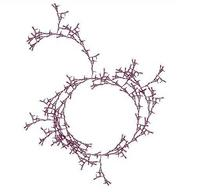University of Virginia, Department of Computer Science CS200: Computer Science, Spring 2003

Notes: Wednesday 19 March 2003

• Today: Read GEB, Aria with Diverse Variations and Chapter 13. This chapter proves that the halting problem is not decidable, and introduces the Church-Turing Thesis (which we will explore more in Classes 32-36). You will not be assigned to read Chapter XIV, but it goes into more depth on Gödel's proof and it recommended.
• Monday, 24 March: Problem Set 6.
Upcoming lab hours:    Wednesday, 19 March: 8-9:30 PM (Jacques)
Thursday, 20 March: 8-9:30 PM (Jacques)
Sunday, 23 March: 1-2:30 PM (Katie)
Sunday, 23 March: 2-3:30 PM (Rachel)
• Monday, 31 March: Problem Set 8 team requests (see Notes 21 for details)

Permute Sort
```(define (flat-one lst)
(if (null? lst) lst (append (car lst) (flat-one (cdr lst)))))

(define (all-permutations lst)
(flat-one
(map
(lambda (n)
(if (= (length lst) 1)
(list lst) ;; Only one permutation of 1-length list
(map
(lambda (oneperm) (cons (nth lst n) oneperm))
(all-permutations (exceptnth lst n)))))
(intsto (length lst)))))

(define (is-sorted? cf lst)
(or (null? lst) (= 1 (length lst))
(and (cf (car lst) (cadr lst))
(is-sorted? cf (cdr lst)))))

(define (permute-sort cf lst)
(car
(filter (lambda (lst) (is-sorted? cf lst))
(all-permutations lst))))
```
Notes

How much work is permute-sort?

What is a problem?

Upper bound O ("big-oh"): f(x) is O (g (x)) means there is a positive constant c such that c * f(x) < g(x) for all but a finite number of x values.

• x2 is O (x2) — c = .5 works fine
• x2 is O (x3) — c = 1 works fine
• x3 is not O (x2) — for any choice of c, once x gets big enough c * x3 > x2
• Time to do bubblesort for a list of length n is O (n2) — the value of c depends on how fast our computer is
• Time to solve the pegboard puzzle with n pegs is O (2n) — we know how to solve it in c * 2n time for some constant c by trying all possible moves (assuming 2 possible moves at each step).
• Time to pegboard puzzle might or might not be O (n) — we don't know of an O (n) procedure to solve it, but we have not proven one does not exist either.
Lower bound Ω ("omega"): f(x) is Ω (g (x)) means there is a positive constant c such that c * f(x) > g(x) for all but a finite number of x values.
• x2 is Ω (x2) — c = 2 works fine
• x2 is not Ω (x3) — for any choice of c, once x gets big enough c * x2 < x3
• x3 is Ω (x2) — for any choice of c, once x gets big enough c * x3 > x2
• Time to do bubblesort for a list of length n is Ω (n2) — the value of c depends on how fast our computer is.
• Time to solve pegboard puzzle with n pegs is might be or might not be Ω (2n) — we don't know if there is a solution to the pegboard puzzle that is faster than c * 2n.
• Time to solve pegboard puzzle is Ω (n) — we do know that there is no solution to the pegboard puzzle that is faster than c * n since any solution involves at least as many moves as their are pegs.
Tight bound Θ ("theta"): f(x) is Θ (g (x)) means that f(x) is O(g (x)) and f(x) is Ω (g (x)).
• x2 is Θ (x2) since x2 is O (x2) and x2 is Ω (x2).
• x2 is not Θ (x3) since x2 is not Ω (x3)
• x3 is not Θ (x2) since x3 is not O (x2)
• Time to do bubblesort for a list of length n is Θ (n2) since it is O (n2) and Ω (n2)
• Time to solve pegboard puzzle with n pegs might or might not be Θ (2n) since it is O (2n) but we don't know if it is Ω (2n) .
• Time to solve pegboard puzzle might or might not be Θ (n) since it is Ω (n) but we don't know it it is O (n) but have not proven it cannot be O (n).
What does it really mean to say a problem is O (n2)?

What does it really mean to say a problem is Ω (n2)?

What does it mean to say a problem is in complexity class P?

What does it mean to say a problem is in complexity class NP?

Are all problems in P also in NP?

Are all problems in NP also in P?

Note: this is the Millennium Prize Problem: The P versus NP Problem. Clay Mathematics Institute will give you \$1M if you answer it, so you may to use the margins to answer it.CS 200: Computer Science Department of Computer Science University of Virginia Circle Fractal by Ramsey Arnaoot and Qi Wang cs200-staff@cs.virginia.edu Using these Materials